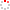CIOMP OpenIR
 Self-consistent adjoint analysis for topology optimization of electromagnetic waves Deng, Y. B.; Korvink, J. G. 2018 Source Publication Journal of Computational PhysicsISSN 0021-9991 Volume 361Pages:353-376 Abstract In topology optimization of electromagnetic waves, the Gateaux differentiability of the conjugate operator to the complex field variable results in the complexity of the adjoint sensitivity, which evolves the original real-valued design variable to be complex during the iterative solution procedure. Therefore, the self-inconsistency of the adjoint sensitivity is presented. To enforce the self-consistency, the real part operator has been used to extract the real part of the sensitivity to keep the real-value property of the design variable. However, this enforced self-consistency can cause the problem that the derived structural topology has unreasonable dependence on the phase of the incident wave. To solve this problem, this article focuses on the self-consistent adjoint analysis of the topology optimization problems for electromagnetic waves. This self-consistent adjoint analysis is implemented by splitting the complex variables of the wave equations into the corresponding real parts and imaginary parts, sequentially substituting the split complex variables into the wave equations with deriving the coupled equations equivalent to the original wave equations, where the infinite free space is truncated by the perfectly matched layers. Then, the topology optimization problems of electromagnetic waves are transformed into the forms defined on real functional spaces instead of complex functional spaces; the adjoint analysis of the topology optimization problems is implemented on real functional spaces with removing the variational of the conjugate operator; the self-consistent adjoint sensitivity is derived, and the phase-dependence problem is avoided for the derived structural topology. Several numerical examples are implemented to demonstrate the robustness of the derived self-consistent adjoint analysis. (C) 2018 Elsevier Inc. All rights reserved. Keyword Topology optimization Self-consistent adjoint sensitivity Electromagnetic wave Perfectly matched layers perfectly matched layer mixed finite-elements level set method shape optimization lie symmetries design equations fluids Computer Science Physics DOI 10.1016/j.jcp.2018.01.045 Indexed By SCI Citation statistics Document Type 期刊论文 Identifier http://ir.ciomp.ac.cn/handle/181722/60803 Collection 中国科学院长春光学精密机械与物理研究所 Recommended CitationGB/T 7714 Deng, Y. B.,Korvink, J. G.. Self-consistent adjoint analysis for topology optimization of electromagnetic waves[J]. Journal of Computational Physics,2018,361:353-376. APA Deng, Y. B.,&Korvink, J. G..(2018).Self-consistent adjoint analysis for topology optimization of electromagnetic waves.Journal of Computational Physics,361,353-376. MLA Deng, Y. B.,et al."Self-consistent adjoint analysis for topology optimization of electromagnetic waves".Journal of Computational Physics 361(2018):353-376.